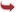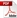International Journal of Scientific and Research Publications

#### IJSRP, Volume 5, Issue 7, July 2015 Edition [ISSN 2250-3153]Analyzing the Response of an RLC Circuit
Sonam Parihar
Abstract: RLC circuit response and analysis is modelled using MATLAB. We calculate the stability of system using bode plot method. Bode plot is one of the convenient method for stability calculation since using it we not only calculate stability but also phase margin ,gain margin, phase crossover frequency, gain crossover frequency at a time. The response of RLC is examined from different input form using MATLAB.
[VIEW FULL PAPER][DOWNLOAD]

Reference this Research Paper (copy & paste below code):

Sonam Parihar (2018); Analyzing the Response of an RLC Circuit; Int J Sci Res Publ 5(7) (ISSN: 2250-3153). http://www.ijsrp.org/research-paper-0715.php?rp=P434288